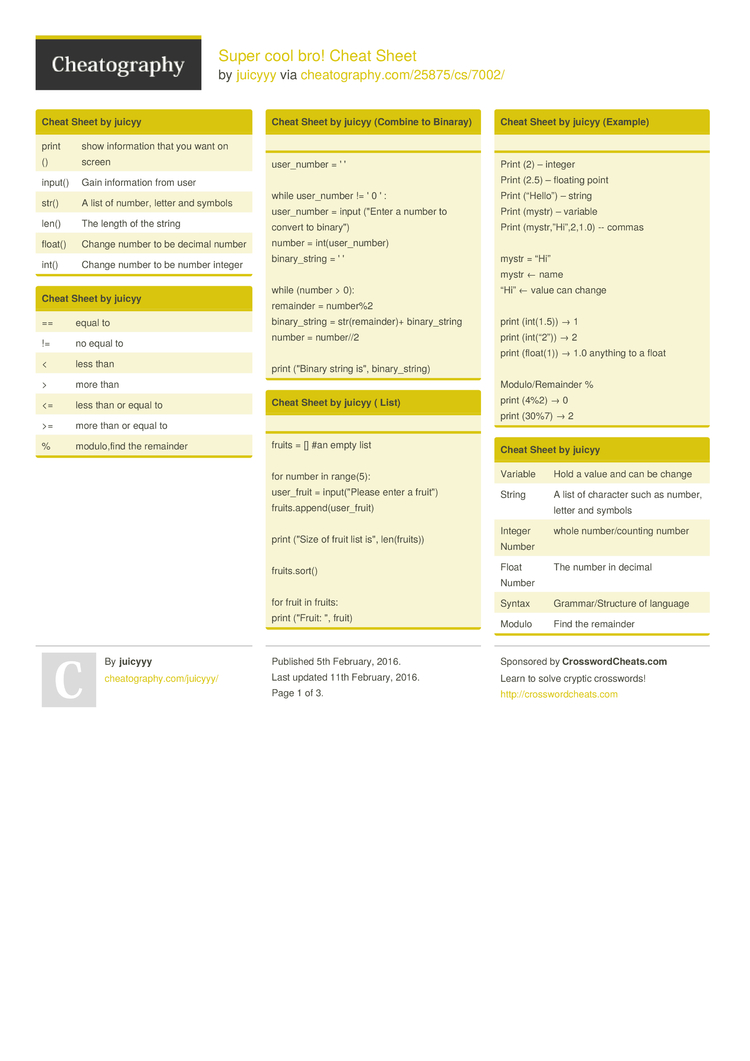Super cool bro! Cheat Sheet by juicyyy

"Come and Say Hi"

Cheat Sheet by juicyy (Combine to Binaray)

user_n­­umber = ' '

while user_n­­umber != ' 0 ' :
user_n­­umber = input ("Enter a number to convert to binary­­")
number = int(us­­er­_­n­umber)
binary­­_s­tring = ' '

while (number > 0):
remainder = number%2
binary­­_s­tring = str(re­­ma­i­n­der)+ binary­­_s­tring
number = number//2

print ("Binary string is", binary­­_s­t­ring)

Cheat Sheet by juicyy ( List)

fruits = [] #an empty list

for number in range(5):
user_fruit = input(­­"­P­lease enter a fruit")
fruits.ap­­pe­n­d­(u­­ser­­_f­ruit)

print ("Size of fruit list is", len(fr­­uits))

fruits.sort()

for fruit in fruits:
print ("Fruit: ", fruit)

Cheat Sheet by juicyy (Example)

Print (2) – integer
Print (2.5) – floating point
Print (“Hello”) – string
Print (mystr) – variable
Print (mystr­­,”­H­i­”,­­2,1.0) -- commas

mystr = “Hi”
mystr ← name
“Hi” ← value can change

print (int(1.5)) → 1
print (int(“2”)) → 2
print (float(1)) → 1.0 anything to a float

Modulo­­/R­e­m­ainder %
print (4%2) → 0
print (30%7) → 2

Cheat Sheet by juicyy

 print () show inform­ation that you want on screen input() Gain inform­ation from user str() A list of number, letter and symbols len() The length of the string float() Change number to be decimal number int() Change number to be number integer

Cheat Sheet by juicyy

 == equal to != no equal to < less than > more than <= less than or equal to >= more than or equal to % modulo­,find the remainder

Cheat Sheet by juicyy ( Naming Conven­tion)

Rule for giving name
- letter
- numbers
- underscore _

Valid name
- _myStr
- my3
- Hello_­­there

Invalid name
- first name=”hi”
- first-name
- first+name

Cheat Sheet by juicyy (Print Name)

name = "tim GIRARD­­"

print (name.u­­p­p­er()) → TIM GIRARD
print (name.l­­o­w­er()) → tim girard
print (name.c­­a­p­i­ta­­lize()) → Tim girard
print (name.t­­i­t­le()) → Tim Girard

Cheat Sheet by juicyy ( Circle)

"­"­"
Python Intro Assignment #2
name
student number
"­­"­"

#Convert the given radius to a floating point

#Make a variable called pi
pi = float(­­3.1­415)

#Calculate the area of the circle using exponents
area = pi(ra­­diu­­s*2)

#Display the area of the circle to the user
print ("The area of the circle is", area)

Cheat Sheet by juicyy

 Variable Hold a value and can be change String A list of character such as number, letter and symbols Integer Number whole number­/co­unting number Float Number The number in decimal Syntax Gramma­r/S­tru­cture of language Modulo Find the remainder Boolean True/False

Cheat Sheet by juicyy

 string­+number Combine together string­+number Crash! number­+number Addition (Math)

Cheat Sheet by juicyy (Reverse)

 ```while True: word = input(­"­Please enter a word") index = 0 reverse = ' ' while int(index) < len(word): reverse = word[i­ndex] + (reverse) index = int(index) + 1 print ("Re­verse: ", reverse)```

Cheat Sheet by juicyy ( Countdown)

 ```user_n­umber = input(­"What number do you want to count down? ") number = int(us­er_­number) countd­own­_string = ' ' while number > 0:       countd­own­_number = countd­own­_string + str(nu­mber) + " "       number = number - 1       #print­(nu­mber) print (count­dow­n_s­tring)```

Cheat Sheet by juicyy ( Line)

mystr = "­­He­l­l­o"

letter_num = 0

while letter_num < len(my­­str):
print (mystr­­[l­e­t­te­­r_num])
letter_num = letter_num + 1
H
e
l
l
o

Cheat Sheet by juicyy

 string­*number Combine that string string­*string Crash! number­*number Multiply (Math) string­**s­tring Crash! number­**n­umber Exponent (Math) string­**n­umber Crash!3 Pages
//media.cheatography.com/storage/thumb/juicyyy_super-cool-bro.750.jpg

PDF (recommended)